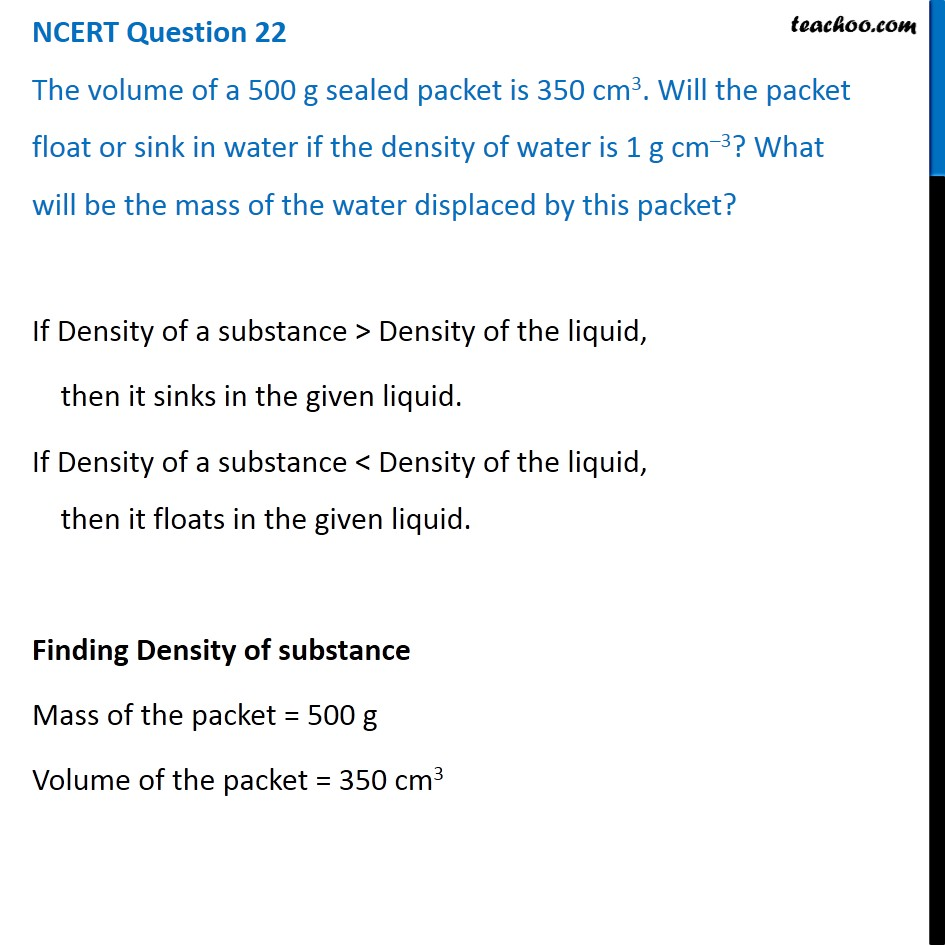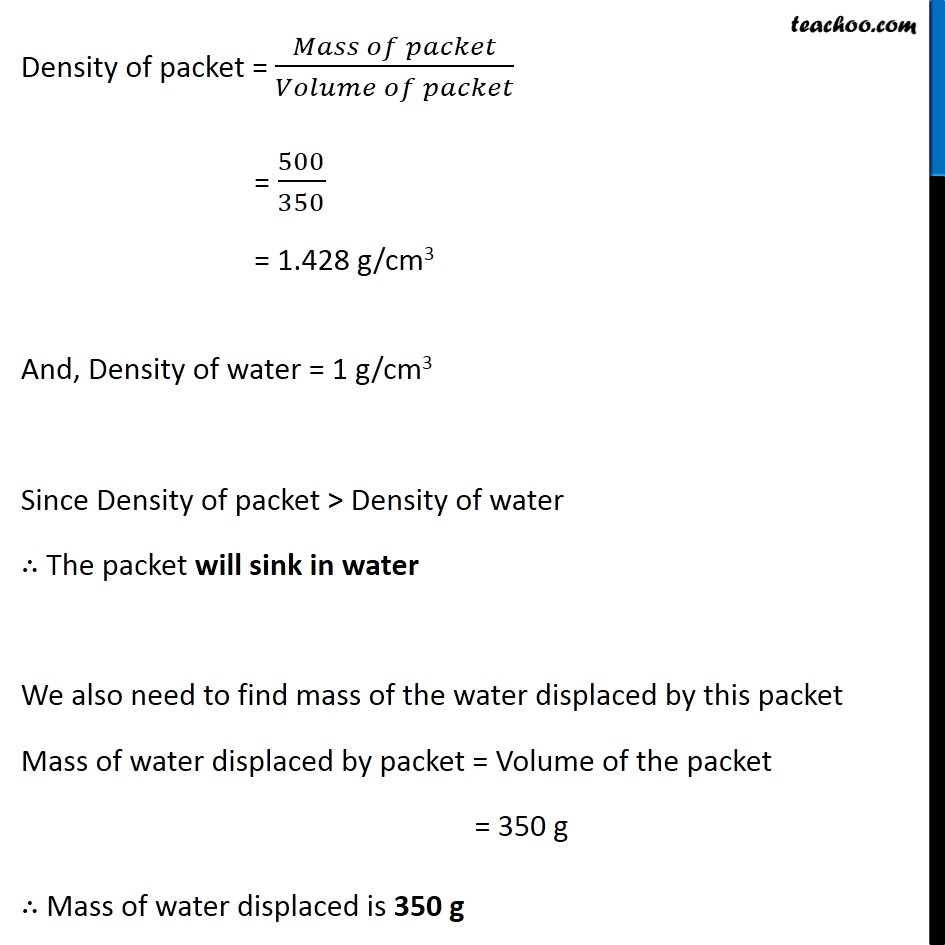NCERT Questions

Class 9
Chapter 10 Class 9 - GravitationLearn in your speed, with individual attention - Teachoo Maths 1-on-1 Class

### Transcript

NCERT Question 22 The volume of a 500 g sealed packet is 350 cm3. Will the packet float or sink in water if the density of water is 1 g cm–3? What will be the mass of the water displaced by this packet? If Density of a substance > Density of the liquid, then it sinks in the given liquid. If Density of a substance < Density of the liquid, then it floats in the given liquid. Finding Density of substance Mass of the packet = 500 g Volume of the packet = 350 cm3 Density of packet = (𝑀𝑎𝑠𝑠 𝑜𝑓 𝑝𝑎𝑐𝑘𝑒𝑡)/(𝑉𝑜𝑙𝑢𝑚𝑒 𝑜𝑓 𝑝𝑎𝑐𝑘𝑒𝑡) = 500/350 = 1.428 g/cm3 And, Density of water = 1 g/cm3 Since Density of packet > Density of water ∴ The packet will sink in water We also need to find mass of the water displaced by this packet Mass of water displaced by packet = Volume of the packet = 350 g ∴ Mass of water displaced is 350 g